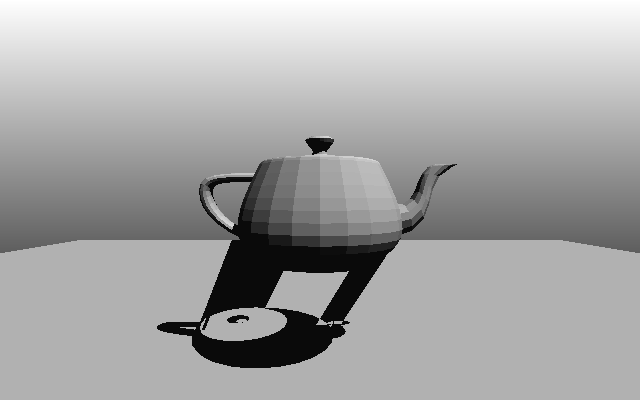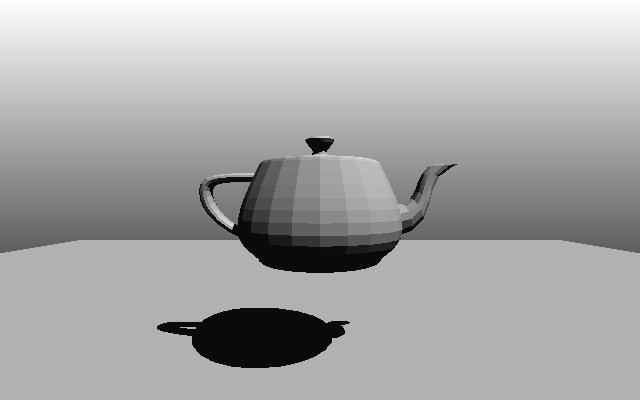[^] [@]

# (Mostly) Robust Stencil Shadow Volumes

#### (posted 2019-10-14; updated 2020-09-13)

Screen coverage of a 3D scene's shadow can be calculated by enclosing the shaded region in a special volume mesh and then rasterizing this mesh in a certain way to a dedicated stencil buffer. After all the volume meshes in the scene have been calculated and rendered, the stencil buffer contains certain values wherever a light affects or does not affect a pixel. The creation of this volume mesh involves finding certain edges between triangles in the source mesh, extruding these edges away from the light source towards infinity, and creating quadrilaterals between these edges and their extruded counterparts. Depending on the rasterization technique used, additional so-called 'cap' geometry, which is constructed by selecting certain input triangles and their extruded versions, is also required.

The classical method for constructing shadow volume meshes is to try to figure out which edges form the so-called 'silhouette' that separates the surfaces of the source mesh that face the light and those that face away from it and then extrude those edges. An extensive description of this technique can be read here (link goes to gamasutra.com). One issue with this approach is that only a certain class of meshes (those where every edge is shared by exactly two triangles) may cast shadows or else visual artifacts will appear.(The teapot model contains some edges that have only one triangle neighbor. The classical technique only works for edges with two neighbor triangles.)

### A robust algorithm

Another technique that always produces correct results exists, though: simply extrude and render every edge in the source mesh. The major downside of this (possibly among other things) is that fillrate and performance requirements can increase extremely.

It may become apparent that not every edge needs to be extruded: some neighboring edges should cancel each other out. For this, we keep track of how often each edge is visited, as if it were rendered -- each time an edge is visited, an associated edge render count (a signed integer) is updated. After considering every edge of every triangle, we know exactly how often each edge needs to be extruded/drawn, likely not at all. Additionally, this technique also handles other edge topologies, like many triangles sharing a single edge, nicely.

A description of this algorithm is as follows:

1. Have a temporary buffer called edge_count with enough space for one signed integerN1 for each possible edge available.
2. Initialize all elements in edge_count to zero.
3. For each triangle t in the input mesh that has the correct orientationN2 towards the light source:
1. If cap geometry is required: append the triangles {ta, tb, tc} and {sc, sb, sa} formed by the vertices a, b, and c of t and its extrusion s to the output mesh.N3
2. For each edge e in t:
1. Find the edge_count buffer index id that uniquely identifies e.N4
2. Compare the indices a and b of the two vertices that make up e.
3. If a < b then increment edge_count[id].
4. If a > b then decrement edge_count[id].
4. For each edge e that may have been visited in section III.b.:
1. Find the buffer index id in edge_count that corresponds to e.N4
2. While edge_count[id] is smaller than zero:
1. Append the quadrilateral {eb, fb, fa, ea} formed by the vertices a and b of e and its extrusion f to the output mesh.N3
2. Increment edge_count[id].
3. While edge_count[id] is larger than zero:
1. Append the quadrilateral {ea, fa, fb, eb} formed by the vertices a and b of e and its extrusion f to the output mesh.N3
2. Decrement edge_count[id].
5. Rasterize the volume mesh the same way as with the classical algorithm.

N1The integer can be of very limited range: only the range of all the edges that meet needs to be stored here. An 8-bit quantity should be more than enough. For input meshes where every edge neighbors exactly two triangles, only 1 bit should suffice.
N2Orientation is in this case defined as the dot product between the triangle's plane equation and the light's position. The algorithm only supports casting shadows from triangles that either face the light or face away from it but not both at the same time, hence only mostly robust volumes.
N3Care should be taken of the winding of these triangles and quadrilaterals: they need to always either point inside or outside of the shadow volume.
N4This is the slowest part of the algorithm. Luckily, the edges and their associated vertices in the input mesh can be precomputed.(Most artifacts are gone but surfaces that do not face the light source still do not cast a shadow.)

### Performance and other considerations

• The input mesh should be composed of triangles with indexed vertices. If this is not the case, the algorithm will have similar performance characteristics as the naive robust version, thus becoming entirely pointless.
• The edge connectivity should be precomputed to speed up sections III.a.2. and IV.a.
First, a list containing each possible unique edge in the source mesh must be created. Each edge in this list is represented by two vertex indices. The ordering of these indices shouldn't matter; edge {a, b} is considered the same as edge {b, a}. Each edge should only appear once in this list. With this list, each edge in section IV can be directly indexed.
Second, each triangle needs to be associated with its three neighboring edges. Since we already have a list containing each edge, we compare each edge of each triangle with the list and store the three associated edge indices together with the triangle. With this information, the edge list can be directly indexed in section III.b.1 from the triangle.
• This algorithm usually generates more geometry than the classical version, even for meshes that are compatible with both versions. The classical algorithm is usually more performant.
• Using the triangles facing away from the light source (as opposed to those facing the light source) for volume creation seems to be the more useful approach for most practical scenarios.